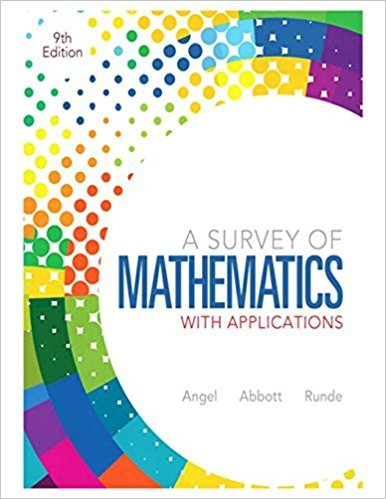×
×

# Solutions for Chapter 12.4: Probability## Full solutions for A Survey of Mathematics with Applications | 9th Edition

ISBN: 9780321759665Solutions for Chapter 12.4: Probability

Solutions for Chapter 12.4
4 5 0 404 Reviews
31
1
##### ISBN: 9780321759665

Chapter 12.4: Probability includes 59 full step-by-step solutions. This expansive textbook survival guide covers the following chapters and their solutions. Since 59 problems in chapter 12.4: Probability have been answered, more than 70856 students have viewed full step-by-step solutions from this chapter. A Survey of Mathematics with Applications was written by and is associated to the ISBN: 9780321759665. This textbook survival guide was created for the textbook: A Survey of Mathematics with Applications, edition: 9.

Key Math Terms and definitions covered in this textbook
• Associative Law (AB)C = A(BC).

Parentheses can be removed to leave ABC.

• Basis for V.

Independent vectors VI, ... , v d whose linear combinations give each vector in V as v = CIVI + ... + CdVd. V has many bases, each basis gives unique c's. A vector space has many bases!

• Big formula for n by n determinants.

Det(A) is a sum of n! terms. For each term: Multiply one entry from each row and column of A: rows in order 1, ... , nand column order given by a permutation P. Each of the n! P 's has a + or - sign.

• Complex conjugate

z = a - ib for any complex number z = a + ib. Then zz = Iz12.

• Cross product u xv in R3:

Vector perpendicular to u and v, length Ilullllvlll sin el = area of parallelogram, u x v = "determinant" of [i j k; UI U2 U3; VI V2 V3].

• Echelon matrix U.

The first nonzero entry (the pivot) in each row comes in a later column than the pivot in the previous row. All zero rows come last.

• Fibonacci numbers

0,1,1,2,3,5, ... satisfy Fn = Fn-l + Fn- 2 = (A7 -A~)I()q -A2). Growth rate Al = (1 + .J5) 12 is the largest eigenvalue of the Fibonacci matrix [ } A].

• Hermitian matrix A H = AT = A.

Complex analog a j i = aU of a symmetric matrix.

• Identity matrix I (or In).

Diagonal entries = 1, off-diagonal entries = 0.

• Inverse matrix A-I.

Square matrix with A-I A = I and AA-l = I. No inverse if det A = 0 and rank(A) < n and Ax = 0 for a nonzero vector x. The inverses of AB and AT are B-1 A-I and (A-I)T. Cofactor formula (A-l)ij = Cji! detA.

• Linear transformation T.

Each vector V in the input space transforms to T (v) in the output space, and linearity requires T(cv + dw) = c T(v) + d T(w). Examples: Matrix multiplication A v, differentiation and integration in function space.

• Orthogonal subspaces.

Every v in V is orthogonal to every w in W.

• Pascal matrix

Ps = pascal(n) = the symmetric matrix with binomial entries (i1~;2). Ps = PL Pu all contain Pascal's triangle with det = 1 (see Pascal in the index).

• Rank one matrix A = uvT f=. O.

Column and row spaces = lines cu and cv.

• Reflection matrix (Householder) Q = I -2uuT.

Unit vector u is reflected to Qu = -u. All x intheplanemirroruTx = o have Qx = x. Notice QT = Q-1 = Q.

• Right inverse A+.

If A has full row rank m, then A+ = AT(AAT)-l has AA+ = 1m.

• Standard basis for Rn.

Columns of n by n identity matrix (written i ,j ,k in R3).

• Stiffness matrix

If x gives the movements of the nodes, K x gives the internal forces. K = ATe A where C has spring constants from Hooke's Law and Ax = stretching.

• Subspace S of V.

Any vector space inside V, including V and Z = {zero vector only}.

• Transpose matrix AT.

Entries AL = Ajj. AT is n by In, AT A is square, symmetric, positive semidefinite. The transposes of AB and A-I are BT AT and (AT)-I.

×